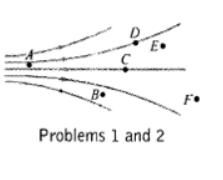# Problem: At which point in the diagram is the magnetic field strength (a) the smallest and (b) the largest? Explain.

###### FREE Expert Solution

The magnetic field strength is smallest where the magnetic field lines are far apart.

On the other hand, the magnetic field strength is largest at the point where the magnetic field lines are closer together.

91% (427 ratings)###### Problem Details

At which point in the diagram is the magnetic field strength (a) the smallest and (b) the largest? Explain.